# Texas Go Math Grade 7 Lesson 4.3 Answer Key Similar Shapes and Scale Drawings

Refer to our Texas Go Math Grade 7 Answer Key Pdf to score good marks in the exams. Test yourself by practicing the problems from Texas Go Math Grade 7 Lesson 4.3 Answer Key Similar Shapes and Scale Drawings.

## Texas Go Math Grade 7 Lesson 4.3 Answer Key Similar Shapes and Scale Drawings

Explore Activity 1

Finding Dimensions

Scale drawings and scale models are used in mapmaking, construction, and other trades.

A blueprint is a technical drawing that usually displays architectural plans. Pete’s blueprint shows a layout of a house. Every 4 inches in the blueprint represents 3 feet of the actual house. One of the walls in the blueprint is 24 inches long. What is the actual length of the wall?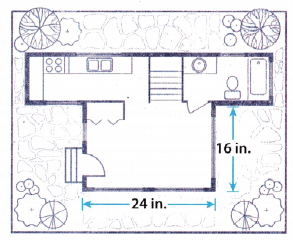A. Complete the table to find the actual length of the wall.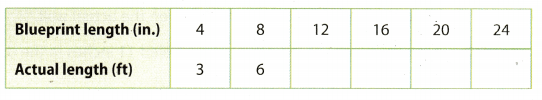Reflect

Question 1.
In Pete’s blueprint the length of a side wall is 16 inches. Find the actual length of the wall.
First, divide by 4 and then multiply by 3 to find the actual length of the wall.
16 ÷ 4 × 3 = 4 × 3
= 12 ft
The actual length of the wall is 12 ft

Question 2.
The back wall of the house is 33 feet long. What is the length of the back wall in the blueprint?
Now, do the opposite. First, divide by 3 and then multiply by 4 to find length of the wall in the blueprint.
33 ÷ 3 × 4 = 11 × 4
= 44 in.
The length of the wall in the blueprint is 44 in.

Question 3.
Check for Reasonableness How do you know your answer to 2 is reasonable?
I know my answer to 2 is reasonable because when I revert the process, I get that the actual length is 33 ft.
44 ÷ 4 × 3 = 11 × 3
= 33 ft.

Example 1

The art class is planning to paint a mural on an outside wall. This figure is a scale drawing of the wall. What is the area of the actual wall?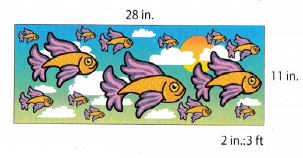STEP 1: Find the number of feet represented by 1 inch in the drawing.
$$\frac{2 \mathrm{in} \cdot \div 2}{3 \mathrm{ft} \div 2}=\frac{1 \mathrm{in}}{1.5 \mathrm{ft}}$$
1 inch in this drawing equals 1.5 feet on the actual wall.

STEP 2: Find the height of the actual wall labeled 11 inches in the drawing.
$$\frac{1 \text { in. } \times 11}{1.5 \mathrm{ft} \times 11}=\frac{11 \mathrm{in} .}{16.5 \mathrm{ft}}$$
The height of the actual wall is 16.5 ft.

STEP 3: Find the length of the actual wall labeled 28 inches in the drawing.
$$\frac{1 \mathrm{in} \cdot \times 28}{1.5 \mathrm{ft} \times 28}=\frac{28 \mathrm{in} .}{42 \mathrm{ft}}$$
The length of the actual wall is 42 ft.

STEP 4: Since area is length times width, the area of the actual wall is 16.5 ft × 42 ft = 693 ft2.

Reflect

Question 4.
Analyze Relationships. Flow could you solve the example without having to determine the number of feet represented by 1 inch?
We could simply multiply the height and length by $$\frac{2 \text { in. }}{3 \mathrm{ft} .}$$.

Question 5.
Find the length and width of the actual room, shown in the scale drawing. Then find the area of the actual room. Round your answer to the nearest tenth.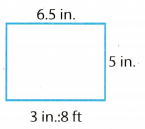Multiply length and width by the scale.
l = 6.5 in. × $$\frac{8 \mathrm{~ft}}{3 \text { in. }}$$
= $$\frac{52}{3}$$
= $$17 . \overline{3}$$ ft

w = 5 in. × $$\frac{8 \mathrm{~ft}}{3 \text { in. }}$$
= $$\frac{40}{3}$$
= $$13 . \overline{3}$$ ft

Now. calculate the area using the following formula: A = l × w
A = l × w
= $$17 . \overline{3}$$ × $$13 . \overline{3}$$
= $$231 . \overline{1}$$ = (round to the nearest tenth) = 231.1 ft2

Question 6.
The drawing plan for an art studio shows a rectangle that is 13.2 inches by 6 inches. The scale in the plan is 3 in.:5 ft. Find the length and width of the actual studio. Then find the area of the actual studio.
Multiply length and width by the scale.
l = 13.2 in. × $$\frac{5 \mathrm{~ft}}{3 \mathrm{~in}}$$
= 22 ft

w = 6 in. × $$\frac{5 \mathrm{ft}}{3 \mathrm{in}}$$
= 10 ft

Now. calculate the area using the following formula: A = l × w
A = l × w
= 22 × 10
= 220 ft2

Explore Activity 2

Drawing in Different Scales
A. A scale drawing of a meeting hail is drawn on centimeter grid paper as shown. The scale is 1 cm:3 m.
Suppose you redraw the rectangle on centimeter grid paper using a scale of 1 cm:6 m. In the new scale, 1 cm represents more than/less than 1 cm in the old scale.
The measurement of each side of the new drawing will be twice/half as long as the measurement of the original drawing.B. Draw the rectangle for the new scale 1 cm:6 m.

Reflect

Question 7.
Find the actual length of each side of the hall using the original drawing. Then find the actual length of each side of the hall using the your new drawing and the new scale. Flow do you know your answers are correct?
First measure length and width on the scale drawing.
l = 4cm
w = 3 cm
Now, find the actual length and width using the scale.
l = 4 × 3 = 12 m
w = 3 × 3 = 9 m
Next find the actual length and width using the scale and new drawing
l = 2 × 6 = 12 m
w = 1.5 × 6 = 9m
I know my answers are correct because I get the same actual length and width using both drawings.

Question 1.
The scale of a room in a blueprint is 3 in : 5 ft. A wall in the same blueprint is 18 in. Complete the table. (Explore Activity 1)Complete the table by multiplying all lengths by the scale.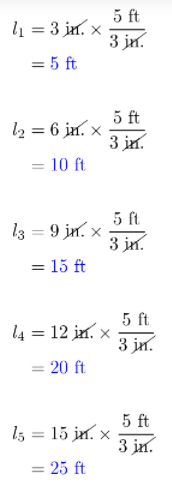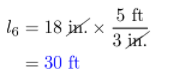a. How long is the actual wall?
Check the table. The actual wall is 30 ft long.

b. A window in the room has an actual width of 2.5 feet. Find the width of the window in the blueprint.
Multiply the width by the scale.
w = 2.5 ft × $$\frac{3 \text { in. }}{5 \mathrm{~ft}}$$
= 1.5 in.
The actual width of the window is 1.5 in.

Question 2.
The scale in the drawing is 2 in.: 4 ft. What are the length and 14 in. width of the actual room? Find the area of the actual room. (Example 1)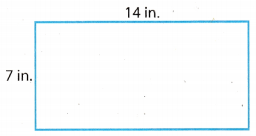Multiply length and width by the scale.
l = 14 in. × $$\frac{4 \mathrm{~ft}}{2 \mathrm{~in} .}$$
= 28 ft

w = 7 in. × $$\frac{4 \mathrm{~ft}}{2 \mathrm{~in} .}$$
= 11 ft
Now. calculate the area using the following formula: A = l × w
A = l × w
= 28 × 11
= 392 ft2

Question 3.
The scale in the drawing is 2 cm : 5 m. What are the length and width 10 cm of the actual room? Find the area of the actual room. (Example 1)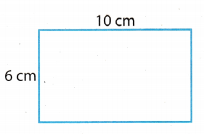Multiply length and width by the scale.
l = 10 cm × $$\frac{5 \mathrm{~m}}{2 \mathrm{~cm}}$$
= 25 m

w = 6 cm × $$\frac{5 \mathrm{~m}}{2 \mathrm{~cm}}$$
= 15 m
Now, calculate the area using the following formula: A = l × w
A = l × w
= 25 × 15
= 375 ft2

Question 4.
A scale drawing of a cafeteria is drawn on centimeter grid paper as shown. The scale is 1 cm : 4 m. (Explore Activity 2)
a. Redraw the rectangle on centimeter grid paper using a scale of 1 cm:6 m.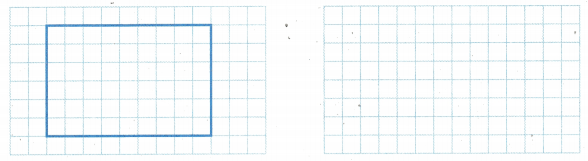First, find length and width on the scale drawing.
l = 4.5 cm
w = 3 cm.
Now, find the actual dimensions and then use scale to find dimensions to redraw the rectangle.
Actual length using original scale length: l = 4.5 cm × $$\frac{4 \mathrm{~m}}{1 \mathrm{~cm}}$$
= 18 m
New scale length using actual length: l = 18m × $$\frac{1 \mathrm{~cm}}{6 \mathrm{~m}}$$
= 3 cm

Actual width using original scale width: w = 3 cm × $$\frac{4 \mathrm{~m}}{1 \mathrm{~cm}}$$
= 12 m
New scale width using actual width: w = 12 m × $$\frac{1 \mathrm{~cm}}{6 \mathrm{~m}}$$
= 2 m
Redraw the rectangle using new scale length and width. (Picture below)

b. What is the actual length and width of the cafeteria using the original scale? What are the actual dimensions of the cafeteria using the new scale?
The actual length and width using original scale and new scale are the same.
l = 18m
w = 12w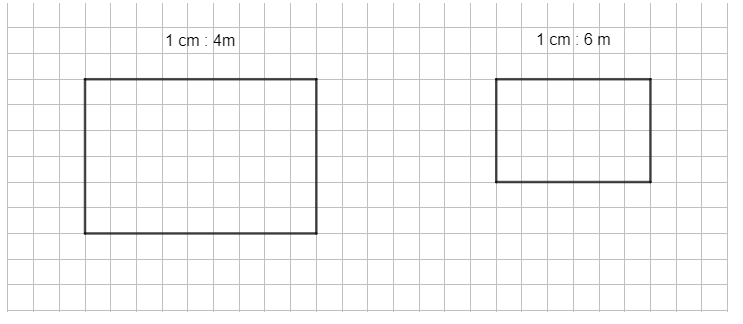Essential Question Check-In

Question 5.
If you have an accurate, complete scale drawing and the scale, which measurements of the object of the drawing can you find?
We can find actual measurements of the object of the drawing.

Question 6.
Art Marie has a small copy of Rene Magritte’s famous painting, The Schoolmaster. Her copy has dimensions 2 inches by 1.5 inches. The scale of the copy is 1 in:40 cm.
a. Find the dimensions of the original painting.
Multiply the dimensions by the scale to get the dimensions of the original painting.
l = 2 in. × $$\frac{40 \mathrm{~cm}}{1 \mathrm{~in} .}$$
= 2 in. × $$\frac{40 \mathrm{~cm}}{1 \mathrm{~in} .}$$
= 80 cm

w = 1.5 in. × $$\frac{40 \mathrm{~cm}}{1 \mathrm{~in} .}$$
= 1.5 in. × $$\frac{40 \mathrm{~cm}}{1 \mathrm{~in} .}$$
= 60 cm

b. Find the area of the original painting.
Calculate the area using the following equation: A = l × w
A = l × w
= 80 × 60
= 4800 cm2

c. Since 1-inch is 2.54 centimeters, find the dimensions of the original painting in inches.
Find the dimensions of the original painting in niches by multiplying the dimensions by the conversion factor.
Conversion factor: $$\frac{1 \mathrm{~in} .}{2.54 \mathrm{~cm}}$$
l = 80 cm × $$\frac{1 \mathrm{~in} .}{2.54 \mathrm{~cm}}$$
= 80 cm × $$\frac{1 \mathrm{~in} .}{2.54 \mathrm{~cm}}$$
≈ 31.5 in.

w = 60 cm × $$\frac{1 \mathrm{~in} .}{2.54 \mathrm{~cm}}$$
= 60 cm × $$\frac{1 \mathrm{~in} .}{2.54 \mathrm{~cm}}$$
≈ 23.6 in.

d. Find the area of the original painting in square inches.
Calculate the are using the following equation: A = l × w
A = l × w
≈ 31.5 × 23.6
≈ 743.4 in.2

Question 7.
A game room has a floor that is 120 feet by 75 feet. A scale drawing of the floor on grid paper uses a scale of 1 unit:5 feet. What are the dimensions of the scale drawing?
Multiply actual dimensions by the scale to get the dimensions of the scale drawing.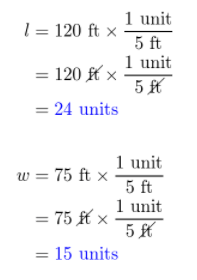Question 8.
Multiple Representations The length of a table is 6 feet. On a scale drawing, the length is 2 inches. Write three possible scales for the drawing.
Divide the length on a scale drawing by the actuaL Length to get the scale.
2 inches : 6 feet
Now, we can write it as a fraction, and expand or simplify to get 2 more possible scales.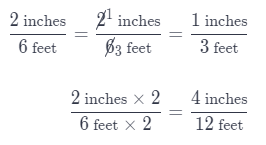We got 2 more possible scales:
1 inches : 3 feet
4 inches : 12 feet

Question 9.
Analyze Relationships A scale for a scale drawing is 10 cm:1 mm. Which is larger, the actual object or the scale drawing? Explain.
The scale drawing is larger, because 10 cm on the scale drawing is 1 mm on the actual object

Question 10.
Architecture The scale model of a building is 5.4 feet tall.
a. If the original building is 810 meters tall, what was the scale used to make the model?
Divide the scale modeL height by the actual height to get the scale used to make the model.
$$\frac{5.4 \text { feet }}{810 \text { meters }}=\frac{5.4 \div 5.4 \text { feet }}{810 \div 5.4 \text { meters }}$$
= $$\frac{1 \text { foot }}{150 \text { meters }}$$
The scale used to make the model was: 1 foot : 150 meters

b. If the model is made out of tiny bricks each measuring 0.4 inch in height, how many bricks tall is the model?
Divide the scale model height by the brick height to get how many bricks talL is the model.
5.4 ÷ 0.4 = 13.5
The scale model is 13.5 bricks tall.

Question 11.
You have been asked to build a scale model of your school out of toothpicks. Imagine your school is 30 feet tall. Your scale is 1 ft:1.26 cm.
a. If a toothpick is 6.3 cm tall, how many toothpicks tall will your model be?
First, find out how many cm is your scale model tall by multiplying actual height by the scale.
h = 30 ft × $$\frac{1.26 \mathrm{~cm}}{1 \mathrm{~ft}}$$
= 30 ft × $$\frac{1.26 \mathrm{~cm}}{1 \mathrm{~ft}}$$
= 37.8 cm
Now, divide obtained scale model height by toothpick height to get how many toothpick tall our scale model will be.
37.8 ÷ 6.3 = 6
Our scale model will be 6 toothpicks tall.

b. Your mother is out of toothpicks, and suggests you use cotton swabs instead. You measure them, and they are 7.6 cm tall. How many cotton swabs tall will your model be?
Divide the scale model height obtained in the subtask above by cotton swabs height to get how many cotton swabs tall our scale model will be.
37.8 ÷ 7.6 ≈ 5
Our scale model will be ≈ 5 cotton swabs tall.

H.O.T. Focus On Higher Order Thinking

Question 12.
Draw Conclusions The area of a square floor on a scale drawing is 100 square centimeters, and the scale of the drawing is 1 centimeter:2 ft, What is the area of the actual floor? What is the ratio of the area in the drawing to the actual area?
First, calculate the length of the floor on a scale drawing using the fact it is a square floor.
A = l2
100 = l2
l = $$\sqrt {100}$$
l = 10 cm

Now, calculate the actual length of the square floor by multiplying the scale drawing length by the scale.
l = 10 cm × $$\frac{2 \mathrm{~ft}}{1 \mathrm{~cm}}$$
= 10 cm × $$\frac{2 \mathrm{~ft}}{1 \mathrm{~cm}}$$
= 20 ft
Next, calculate the actual area of the floor.
A = l2
= 202
= 400 ft2
Write the ratio of the areas.
$$\frac{100 \mathrm{~cm}^{2}}{400 \mathrm{ft}^{2}}=\frac{1 \mathrm{~cm}^{2}}{4 \mathrm{ft}^{2}}$$
The ratio of the area in the drawing to the actual area is 1 : 4.

Question 13.
Multiple Representations Describe how to redraw a scale drawing with a new scale.